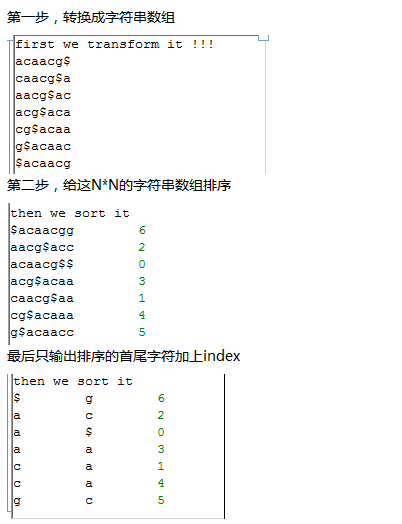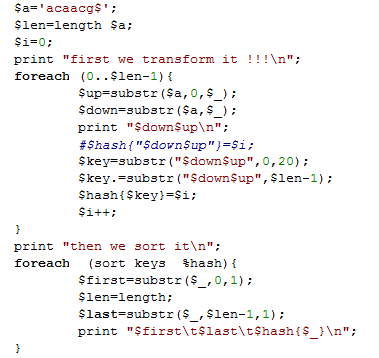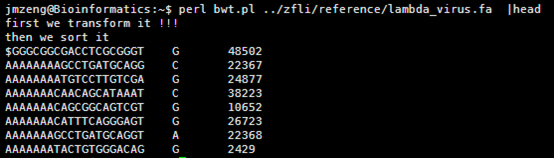# 自己动手写bowtie第一讲：BWT算法详解并建立索引

http://www.cnblogs.com/xudong-bupt/p/3763814.html

A1A2A3...An

A2A3...AnA1

A3...AnA1A2

...

AnA1A2A3...

n个串，每个字符串的长度都是n。Perl程序如下

[perl]

while (<>){

next if />/;

chomp;

\$a.=\$_;

}

\$a.='\$';

\$len=length \$a;

\$i=0;

print "first we transform it !!!\n";

foreach (0..\$len-1){

\$up=substr(\$a,0,\$_);

\$down=substr(\$a,\$_);

#print "\$down\$up\n";

#\$hash{"\$down\$up"}=\$i;

\$key=substr("\$down\$up",0,20);

\$key=\$key.”\t”.substr("\$down\$up",\$len-1);

\$hash{\$key}=\$i;

\$i++;

}

print "then we sort it\n";

foreach  (sort keys  %hash){

\$first=substr(\$_,0,1);

\$len=length;

\$last=substr(\$_,\$len-1,1);

#print "\$first\t\$last\t\$hash{\$_}\n";

print "\$_\t\$hash{\$_}\n";

}

[/perl]http://tieba.baidu.com/p/1504205984

http://www.cnblogs.com/xudong-bupt/p/3763814.html

## One thought on “自己动手写bowtie第一讲：BWT算法详解并建立索引”

1.bwa的算法精髓 on said: